# LTspice: using conditional statements to switch ON & OFF voltage–controlled switches in a resonance circuit

#### frustratedgrape20

Joined Mar 3, 2020
23
Hi,

An initially charged capacitor in a resonance circuit undergoes periodic voltage reversal (blue line), as shown below: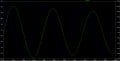A possible circuit to address this problem uses 2 capacitor banks & switch modules, as shown: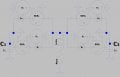C₁ and C₂ represent metallized film capacitor banks of equal capacitance, but the main difference is that the voltage across C₁ will always be ≥0 (0⇔+10 kV) whereas the voltage across C₂ will always be <0.

In order to achieve a sinusoidal decay current waveform, switches SCR₁ through SCR₄ will have to be switched on (via microcontrollers V₁ through V₄) in a particular sequence, according to the following conditions:
· SCR₁: IF (V(C₁)>0 && V(C₂)==0,V₁=4,V₁=1)
· SCR₂: IF (V(C₁)==0 && V(Lᴄᴏɪʟ)<0,V₂=4,V₂=1)
· SCR₃: IF (V(C₁)==0 && V(C₂)<0,V₃=4,V₃=1)
· SCR₄: IF (V(C₁)==0 && V(Lᴄᴏɪʟ)>0,V₄=4,V₄=1)

Basically, here is a quick explanation:
1. upon closing of SCR₁, C₁ discharges until V(C₁)=0
2. SCR₁ turns off and SCR₂ turns on so that current flows through C₂; V(C₂)<0 (e.g. –9.95 kV) when fully charged
3. SCR₂ turns off and SCR₃ turns on
4. C₂ starts discharging through SCR₃ until V(C₃)=0
5. SCR₃ turns off and SCR₄ turns on
6. C₁ is charged by the inductor until V(C₁)≈10 kV (slightly less than +9.95 kV due to circuit resistance)
7. cycle repeats itself

Here is a screenshot of the schematic showing the SPICE directives and comments: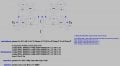A copy of the schematic is attached below. I would like to verify that the above hypothesis is correct and to derive a current waveform through each capacitor bank.

I ran into trouble trying to implement the boolean logic into this schematic, and I would greatly appreciate it anyone who could help with this.

Thanks!

#### Attachments

• 7.5 KB Views: 5
Last edited:

#### LvW

Joined Jun 13, 2013
1,622
Hello
A block diagram of the assembled circuit would be helpful

#### frustratedgrape20

Joined Mar 3, 2020
23
Capacitor bank C₁ is initially charged to 10 kV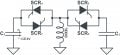SCR₁ closes and C₁ discharges from 10 kV → 0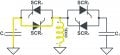At the instance where the voltage across C₁=0 (corresponding to Iₘₐₓ), SCR₁ turns off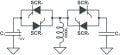At the same time, SCR₂ turns on and allows the collapsing magnetic field to discharge current through C₂, therefore charging C₂ to a negative voltage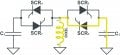When the inductor has fully discharged, C₂ will have been charged to –10 kV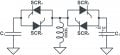SCR₃ immediately closes & C₂ begins discharging from –10 kV → 0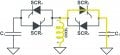When the voltage across C₂=0 (corresponding to Iₘₐₓ through the coil), SCR₃ turns off & SCR₄ turns on simultaneously so that the current flow is uninterrupted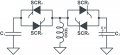The magnetic field in the coil collapses by pulsing current through C₁ and charging it from 0 → 10 kV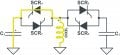SCR₄ turns off when voltage across the coil = 0, and the process repeats itself

Last edited: